Home | | Maths 8th Std | Exercise 1.1: Rational numbers

# Exercise 1.1: Rational numbers

8th Maths : Chapter 1 : Numbers : Text Book Back Exercises Questions with Answers, Solution

Exercise 1.1

Fill in the blanks:

(i) −19/5 lies between the integers __________ and __________. [Answer: −4 and −3]

(ii) The decimal form of the rational number 15/-4 is __________. [Answer: −3.75]

(iii) The rational numbers -8/3 and 8/3 are equidistant from __________. [Answer: 0]

(iv) The next rational number in the sequence 15/24, 20/32 , 25/40 is __________. [Answer: 30/−48]

(v) The standard form of 58/78 is __________. [Answer: −29/39]

2. Say True or False:

(i) 0 is the smallest rational number. [Answer: False]

(ii) −4/5 lies to the left of 3/4. [Answer: True]

(iii) −19/5 is greater than -15/4 . [Answer: False]

(iv) The average of two rational numbers lies between them. [Answer: True]

(v) There are an unlimited number of rational numbers between 10 and 11. [Answer: True]

3. Find the rational numbers represented by each of the question marks marked on the following number lines.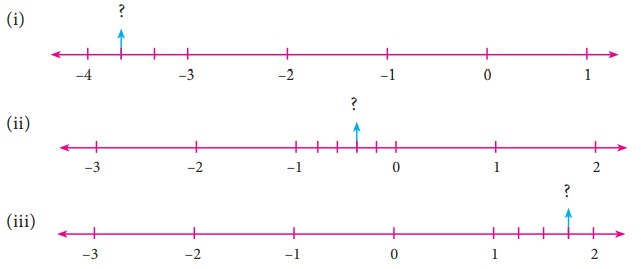Solution:

(i) The number lies between −3 and −4. The unit part between −3 and −4 is divided into 3 equal parts and the second part is asked.

The required number is −3 (2/3) = − 11/3.

(ii) The required number lies between 0 and −1. The unit part between 0 and −1 is divided into 5 equal parts, and the second part is taken.

The required number is −2/5

(iii) The required number lies between 1 and 2. The unit part between 1 and 2 is divided into 4 equal parts and the third part is taken.

The required number is 1 (3/4) = 7/4

4. The points S, Y, N, C, R, A, T, I and O on the number line are such that CN=NY=YS and RA=AT=TI=IO. Find the rational numbers represented by the letters Y, N, A, T and I.Solution:

Y = −2 + (1/3) = [−6 + 1] / 3 = −5/3

N = [−5/3] + [1/3] = [−5 + 1] / 3 = −4/3

RA = AT = TI = IO = 1/4

A = 2 + [1/4] = [8 + 1] / 4 = 9/4

T = 9/4 + 1/4 = [9 + 1] / 4 = 10/4

I = 10/4 + 1/4 = [10 + 1] / 4 = 11/4

5. Draw a number line and represent the following rational numbers on it.Solution:

(i) 9/4 = 2 (1/4)

∴ 9/4 lies between 2 and 3.(ii) −8/3 = −2 (2/3)

−2 (2/3) lies between −2 and −3.(iii) −17/−5 = 3 (2/5)

3 (2/5) lies between 3 and 4 in the number line.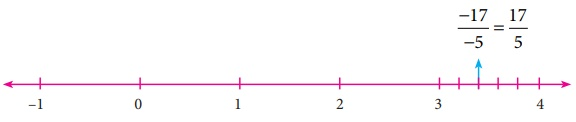(iv) 15/−4 = −3 (3/4)

−3 (3/4) lies between −3 and −4.6. Write the decimal form of the following rational numbers.Solution:

(i) 1/11 = 0.0909…(ii) 13/4 = 3.25(iii) −18/7 = −2.571428571428…(iv) 1 (2/5) = 7/5 = 1.4(v) −3 (1/2) = −7/2 = −3.57. List any five rational numbers between the given rational numbers.Solution:

(i) −2 and 0i.e. −2/1 and 0/1

−2/1 = [−2 × 10] / [1 × 10] = −20/10

0/1 = [0 × 10] / [1 × 10] = 0 / 10

Five rational numbers between −20/10 (= −2) and 0/10 (= 0) are

−20/10, −19/10, −18/10, −7/10, −6/10, −5/10, 0/10 (=0)

(ii) −1/2 and 3/5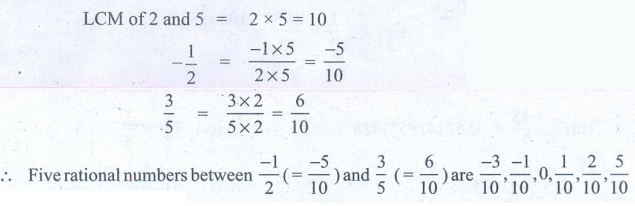LCM of 2 and 5 = 2 × 5 = 10

−1/2  = [−1 × 5] / [2 × 5] = −5 / 10

3/5 = [3 × 2] / [5 × 2] = 6/10

Five rational numbers between −1/2 (= −5/10) and 3/5 (= 6/10) are −3/10, −1/10, 0, 1/10, 2/10, 5/10

(iii) 1/4 and 7/201/4 = [1 × 15] / [4 × 15] = 15/60

7/20 = [7 × 3] / [20 × 3] = 21/60

Five rational numbers between 1/4 (=15/60)  and 7/20 (=21/60) are 16/60, 17/60, 18/60, 19/60, 20/60

(iv) −6 / 4 and −23 / 10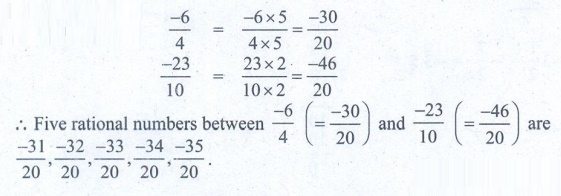−6 / 4 =  [−6 × 5] / [4 × 5] =  −30 / 20

−23 / 10 = [23 × 2] / [10 × 2] = −46 / 20

Five rational numbers between −6/4 ( = −30/20) and −23 / 10 (= −46/20) are −31/20, −32/20, −33/20, −34/20, −35/20.

8. Use the method of averages to write 2 rational numbers between 14/5 and 16/3.

Solution:The average of a and b is 1/2  (a + b)

The average of 14/5 and 16/3 is C1 = 1/2 (14/5 + 16/3)

C1 = 1/2 ([42 + 80] / 15)

C1 = 122/30

C1 = 61/15

14/15 < 61/15 < 16/3            ……….(1)

The average of 14/5 and 61/15 is C2 = 1/2 ( 14/5 + 61/15 )

C2 = 1/2 × ( [42 + 61] / 15)

C2 = 1/2 × 103/15 = 103/30

14/5 < 103/30 < 61/15    …………(2)

From (1), (2) we get, 14/5 < 103/30 < 61/15 < 16/3

9. Compare the following pairs of rational numbers.Solution:

(i) −11/5, −21/8LCM of 5, 8 is 40

−11/5 = [−11 × 8] / [5 × 8] = −88/40

−21/8 = [−21 × 5] / [8 × 5] = −105/40

−105/40 < −88/40

−21/8 < −11/5

(ii) 3/−4, −1/2LCM of 4 and 2 = 4

3/−4 = −3/4

−1/2 = [−1 × 2] / [2 × 2] = −2/4

3/−4 < −2/4

−3/4 < −1/2

(iii) 2/3, 4/5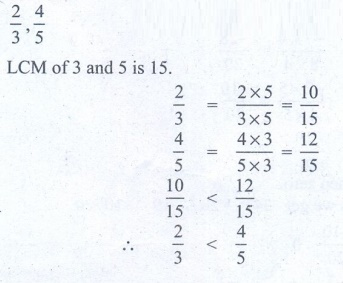LCM of 3 and 5 is 15.

2/3 = [2 × 5] / [3 × 5] = 10 / 15

4/5 = [4 × 3] / [5 × 3] = 12 / 15

10/15 < 12/15

2/3 < 4/5

10. Arrange the following rational numbers in ascending and descending order.(i) −5/−12, −11/8, −15/24, −7/−9, 12/36LCM of 12, 8, 24, 9, 36 is 4 × 3 × 2 × 3 = 72

−5/−12 = [−5 × 6] / [12 × 6] = −30/72

−11/8 = [−11 × 9] / [8 × 9] = −99/72

−15/24 = [−15 × 3] / [24 × 3] = −45/72

−7/−9 = [7 × 8] / [9 × 8] = 56/72

12/36 = [12 × 2] / [36 × 2] = 24/72

Now comparing the numerators −30, −99, −45, 56, 24 we get 56 > 24 > −30 > −45 > −99

i.e 56/72 > 24/72 > −30/72 > −45/72 > −99/72 and so  −7/−9 > 12/36 > −5/12 > −15/24 > −11/8

Descending order −7/−9 >  12/36 > −5/12 > −15/24 > −11/8

Ascending order   −11/8 < −15/24 < −5/12 < 12/36 < −7/−9

(ii) −17/10,  −7/5, 0, −2/4, −19/20LCM of 10, 5, 4, 20 is 5 × 2 × 2 = 20

−17/10 = [−17 × 2] / [10 × 2] = −34/20

−7/5 = [−7 × 4] / [5 × 4] = −28/20

−2/4 = [−2 × 5] / [4 × 5] = −10/20

−19/20 = −19/20

Negative numbers are less then zero.

Arranging the numerators we get −34 < − 28 < −19 < −10 < 0

−34/20 < −28/20 < −19/20 < −10/20 < 0

Ascending order = −17/10 < −7/5 < −19/20 < −2/4 < 0

Descending order 0 > −2/4 > −19/20 > −7/5 > −17/10

Objective Type Questions

11. The number which is subtracted from −6/11 to get 8/9 is __________.[Ans. (B) −142/99 ]

Solution:

Let x be the number to be subtracted

(−6/11) – x = 8/9

(−6 /11) – 8/9 = x

x = [(−6 × 9) + (−8 × 11)] / [11 × 9] =  [−54+ (−88)] /  =  −142 / 99

12. Which of the following pairs is equivalent?Solution:−20/12 =  [−20 ÷ 4] / [12 ÷ 4] = −5/3 ≠ 5/3

16/−30 =  [−16 ÷ 2] / [30 ÷ 2] = −8/15

−18/36 =  [−18 ÷ 9] / [36 ÷ 9] =  −2/4 = [−2 × 11] / [4 × 11] = −22/44 ≠ −20/44

16/−30 and −8/15 are equivalent fraction.

13. -5/4 is a rational number which lies between __________ .Solution:

−5 /4 = − 1 (1/4)

−5/4 lies between −1 and −2.

14. Which of the following rational numbers is the greatest?Solution:LCM of 24, 16, 8, 32 = 8 × 2 × 3 × 2 = 96

−17/24 =  [−17 × 4] / [24 × 4] =   −68/96

−13/16 = [−13 × 6] / [16 × 6] = −78/96

7/−8 = [−7 × 12] / [8 × 12] = −84/96

−31/32 = [−31 × 3] / [32 × 3] = −93/96

−93/96 < −84/96 < −78/96 < −68/96

−31/32 < 7/−8 < −13/16 < −17/24

−17/24 is the greatest number.

15.The sum of the digits of the denominator in the simplest form of 112 / 528 is _________ .

(A) 4

(B) 5

(C) 6

(D) 7

Solution:112/528 = [112 ÷ 8] / [528 ÷ 8] = 14/66 = [14 ÷ 2] / [66 ÷ 2] = 7/33

Sum of digits in the denominator = 3 + 3 = 6

1. (i) –4 and –3 (ii) –3.75 (iii ) 0 (iv) 30/−48 ( v) −29/39

2.(i) False (ii) True (iii) False (iv) True (v) True

3. (i) – 11/3  (ii) −2/5  (iii) 7/4

4. Y = -5/3 , N=-4/3 , A =9/4 , T =10/4 , I =11/4

5. (i)(ii)(iii)(iv)6. (i) 0.0909... (ii) 3.25  (iii) –2.5714285714  (iv) 1.4  (v) –3.5Tags : Questions with Answers, Solution | Numbers | Chapter 1 | 8th Maths , 8th Maths : Chapter 1 : Numbers
Study Material, Lecturing Notes, Assignment, Reference, Wiki description explanation, brief detail
8th Maths : Chapter 1 : Numbers : Exercise 1.1: Rational numbers | Questions with Answers, Solution | Numbers | Chapter 1 | 8th Maths